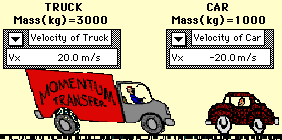## Car and Truck in Head-on Collision

### Inelastic Collision

This animation depicts an inelastic, head-on collision between a truck and a car. In the collision between the truck and the car, total system momentum is conserved. Before the collision, the momentum of the car is +20000 kg*m/s and the momentum of the truck is -60000 kg*m/s; the total system momentum is -4 000 kg*m/s. After the collision, the momentum of the car is -10000 kg*m/s and the momentum of the truck is -30000 kg*m/s; the total system momentum is -40000 kg*m/s. Consistent with the law of conservation of momentum, the total system momentum is conserved. The momentum change of the car (-30000 kg*m/s) is equal in magnitude and opposite in direction to the momentum change of the truck (30000 kg*m/s) .

An analysis of the kinetic energy of the two vehicles reveals that the total system kinetic energy before the collision is 800000 Joules (200000 J for the car plus 600000 J for the truck). After the collision, the total system kinetic energy is 200000 Joules (50000 J for the car and 150000 J for the truck). The total kinetic energybefore the collision is not equal to the total kinetic energy after the collision. A large portion of the kinetic energy is converted to other forms of energy such as sound energy and thermal energy. A collision in which total system kinetic energy is not conserved is known as an inelastic collision.View Mac Version || View Windows Version